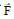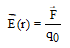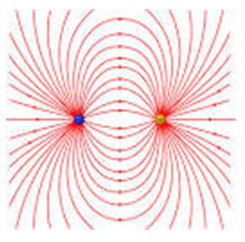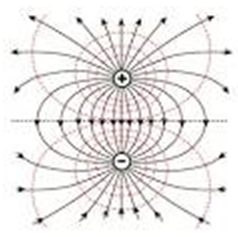# ELECTRIC FIELD AND ELECTRIC POTENTIAL

## ELECTRIC FIELD AND ELECTRIC POTENTIAL

Electric field due to a given charge is defined as the space around the charge in which electrostatic force of attraction or repulsion due to charge can be experienced by any other charge. If a test charge experiences no force at a point, the electric field at that point must be zero.

Electric field intensity at any point is the strength of electric field at that point. It is defined as the force experienced by a unit positive charge placed at that point.

Ifis the force acting on a test charge +q0 at any point r, then electric field intensity at this point is given byElectric field is a vector quantity and its S.I. unit is Newton per coulomb or N/C.

### ELECTRIC POTENTIAL:

The work done in charging a body is stored in it as its electric potential energy. The electric potential energy per unit charge is called electric potential.

Electric Potential = Electric Potential Energy/Charge

Mathematically,

V = W/qSince work is measured in joule and charge in coulomb, therefore electric potential is measured in joule per coulomb (J/C). This unit occurs so often in our study of electricity, so it has been named as volt, in honour of the scientist Alessandra Volta (the inventor of the voltaic cell).

1 Volt = 1Joule/1Coulomb

Potential is a scalar quantity, therefore it is added algebraically. For a positively charged body potential is positive and for a negatively charged body potential is negative.

### ELECTRIC POTENTIAL DIFFERENCE:

Consider a charge Q placed at a point P. Let A and B be two other points (B being closer to A) as shown in figure.

P A B

If a charge q is brought from infinity to A, a work WA will be done.

The potential at A will then be, VA = WA/q

If charge q is brought from infinity to B, the work done will be WB.

The potential at B will then be,  VB = WB/q

The quantity VB-VA is called the potential difference between points A & B in the electric field of charge Q.

Mathematically we have, VB - VA = WB/q - WA/q

Electric potential difference is measured in volt.